# 978-0123869449 Chapter 11 Part 1

Document Type
Homework Help
Book Title
Authors
Michael F. Modest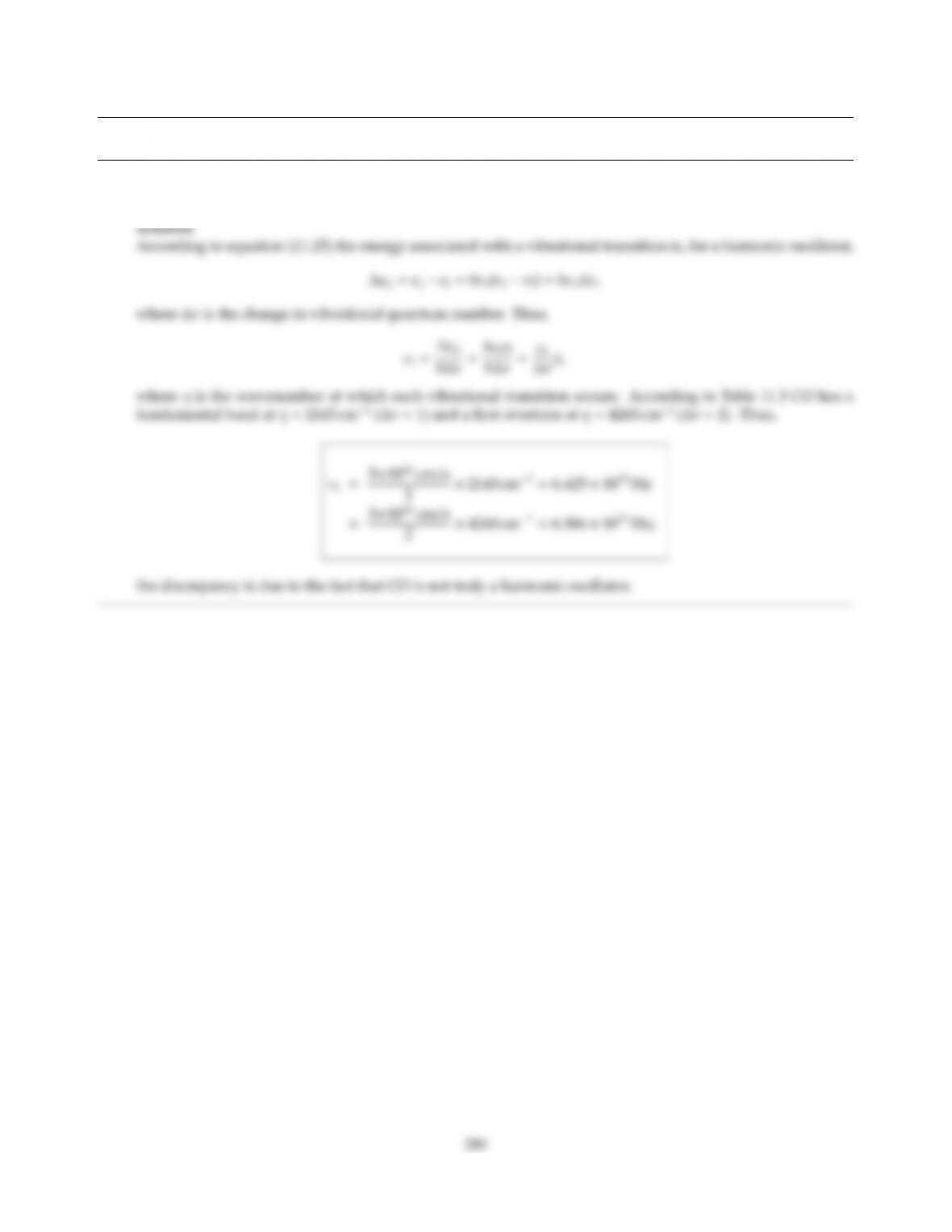CHAPTER 11
11.1 Estimate the eigenfrequency for vibration, νe, for a CO molecule.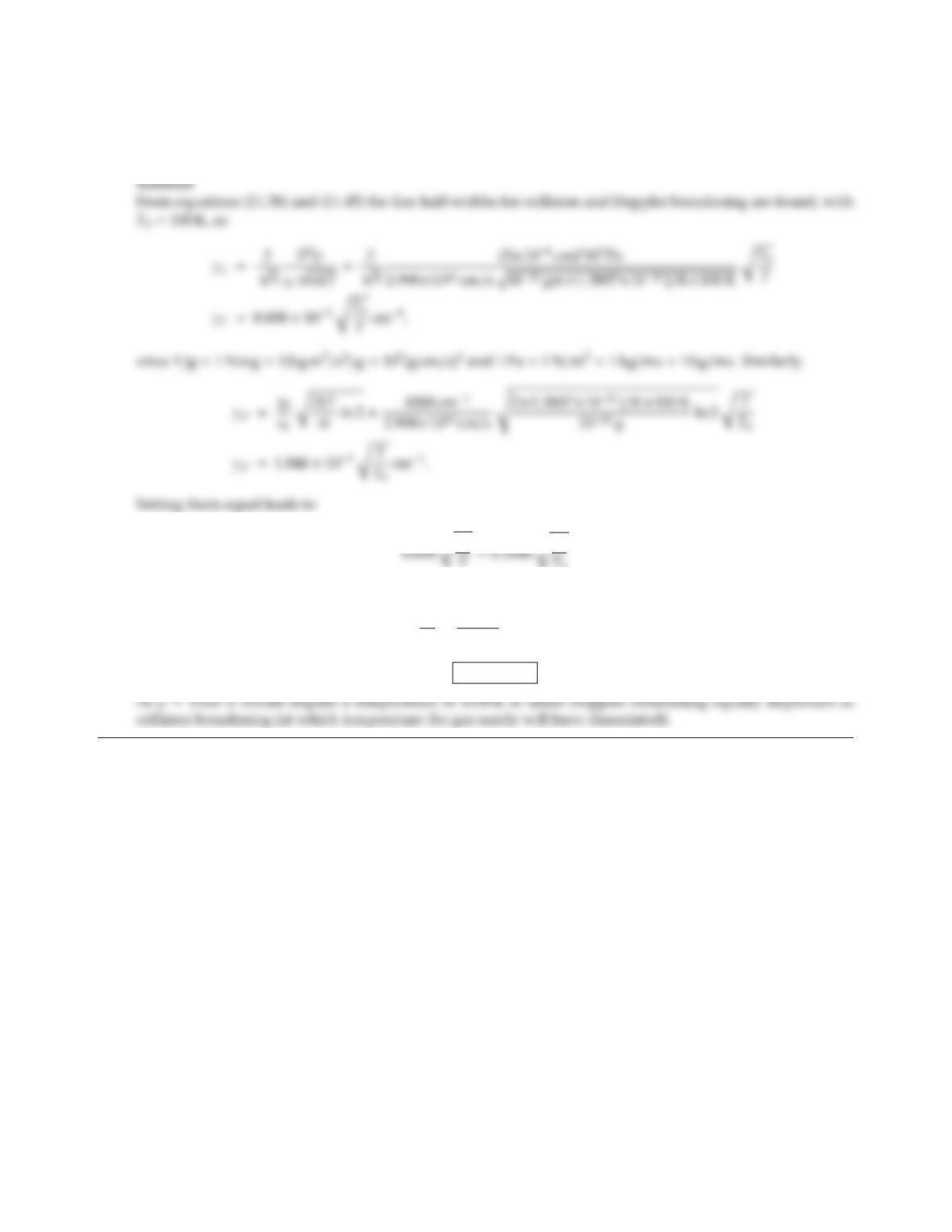11.2 A certain gas at 1 bar pressure has a molecular mass of m=1022 g and a diameter of D=5×108cm. At
what temperature would Doppler and collision broadening result in identical broadening widths for a line at
a wavenumber of 4000 cm1?
T=0.1846 rT
T0
or
T
T0
=8008
0.1846 =43.38
T=4338 K.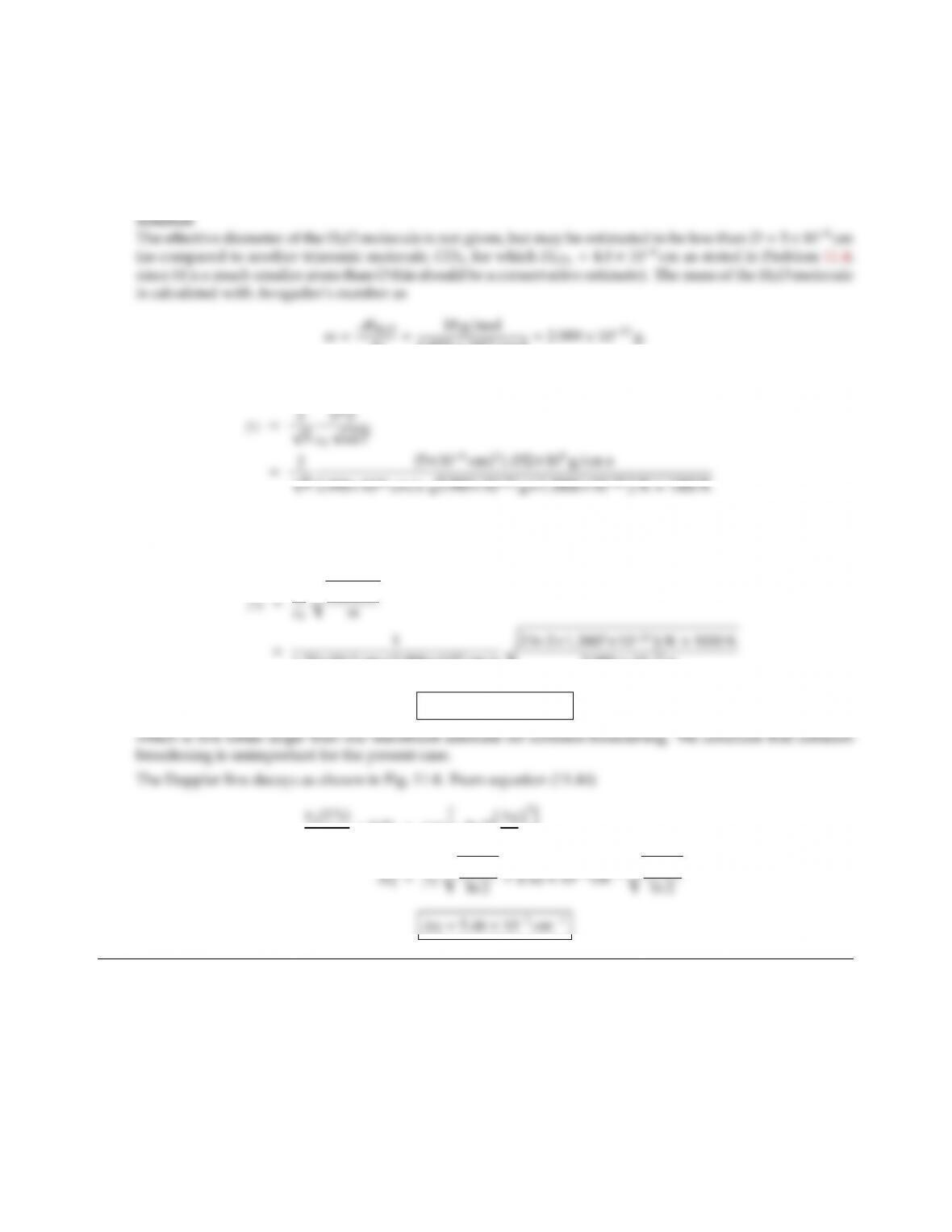CHAPTER 11 291
11.3 Water vapor is known to have spectral lines in the vicinity of λ=1.38 µm. Consider a single, broadened
spectral line centered at λ0=1.33 µm. If the water vapor is at a pressure of 0.1 atm and a temperature of
1000 K, what would you expect to be the main cause for broadening? Over what range of wavenumbers
would you expect the line to be appreciable, i.e., over what range is the absorption coecient at least 1% of
its value at the line center?
NA
6.023 ×1023/mol =2.989 ×1023 g.
Then, from equation (11.38) with 0.1 atm =10.132 kPa =10.132 ×104g/cm s
=4.69 ×103cm1since 1 Jg =1 Nmg =1 kg m2/s2g=107(g cm/s)2.
Similarly, for Doppler broadening from equation (11.45)
1.33×104cm×2.998×1010 cm/ss2 ln 2×1.3807×1023 J/K×1000 K
2.989 ×1023 g
γD=2.12 ×102cm1,
κη0
=0.01 =exp ln 2 η
γD!2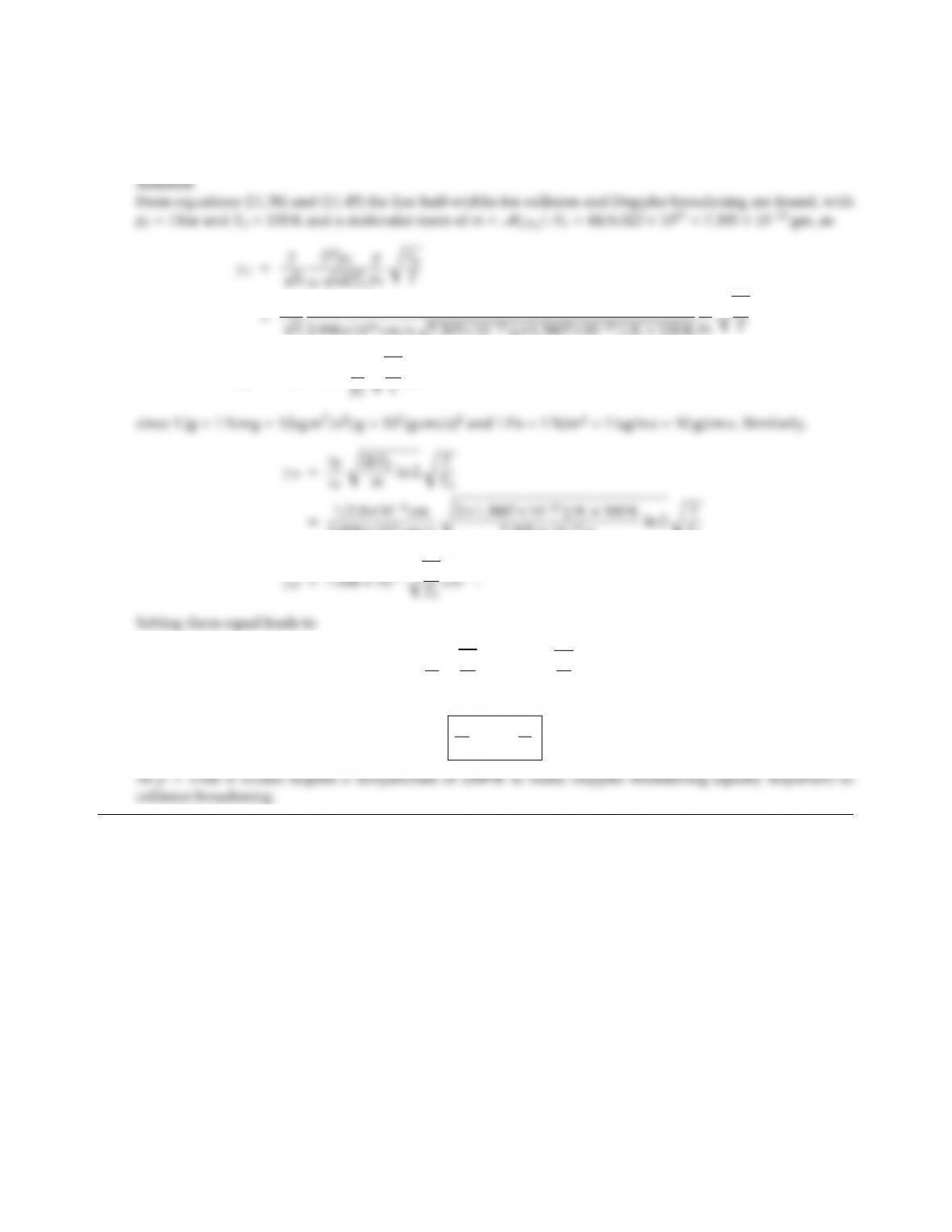11.4 Compute the half-width for a spectral line of CO2at 2.8µm for both Doppler and collision broadening as a
function of pressure and temperature. Find the temperature as function of pressure for which both broadening
phenomena result in the same half-width. (Note: The eective diameter of the CO2molecule is 4.0×108cm).
(4.0×108cm)2×105Pa
p
γC=6.00 ×102p
2.998×1010 cm/ss2×1.3807×1023 J/K×100 K
7.305 ×1023 gln 2 rT
T0
6.00 p
p0rT0
T=0.1928 rT
T0
or
T
T0
=31.1p
p0.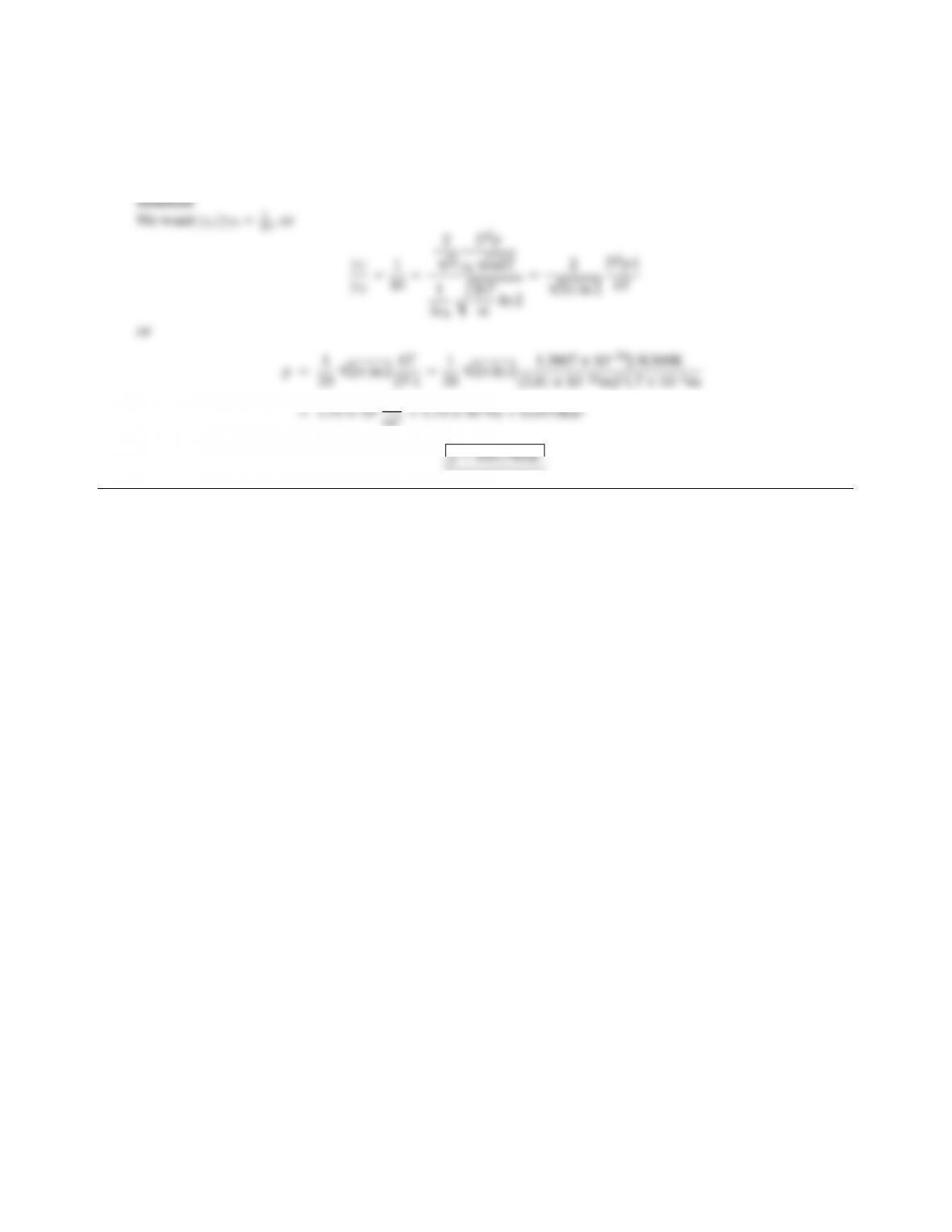CHAPTER 11 293
11.5 Methane is known to have a vibration-rotation band around 1.7 µm. It is desired to measure the Doppler
half-width of a spectral line in that band at room temperature (T=300 K). In order to make sure that collision
broadening is negligible, the pressure of the CH4is adjusted so that the expected collision half-width is only
1/10 of the Doppler half-width. What is this pressure? (For methane: D=0.381 nm.)
m3=1.75 ×103Pa =0.0175bar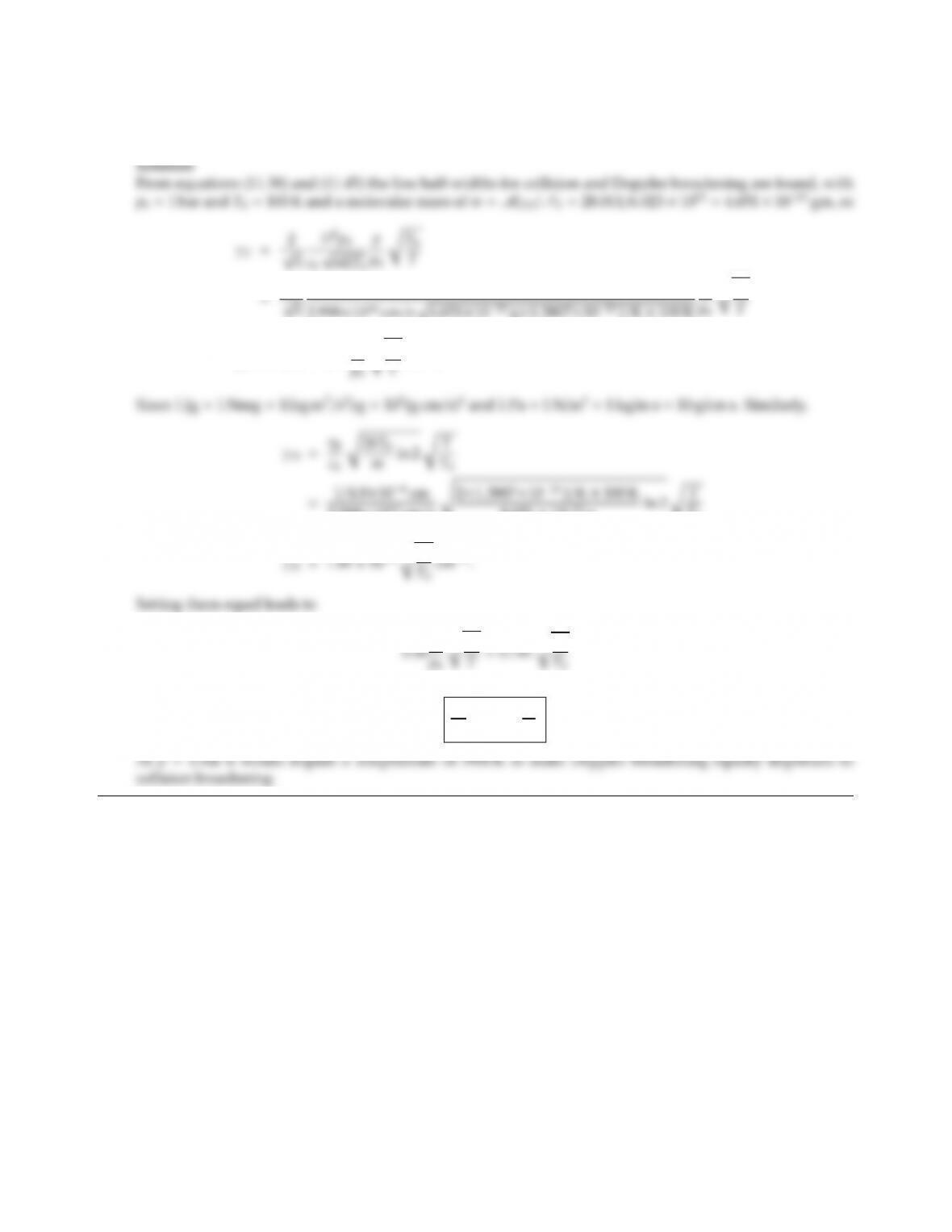11.6 Repeat Problem 11.4 for CO at a spectral location of 4.8µm (Note: The eective diameter of the CO molecule
is 3.4×108cm).
(3.4×108cm)2×1.0132 ×105Pa
p
γC=5.50 ×102p
2.998×1010 cm/ss2×1.3807×1023 J/K×100 K
4.651 ×1023 gln 2 rT
T0
or
T
T0
=39.01 p
p0.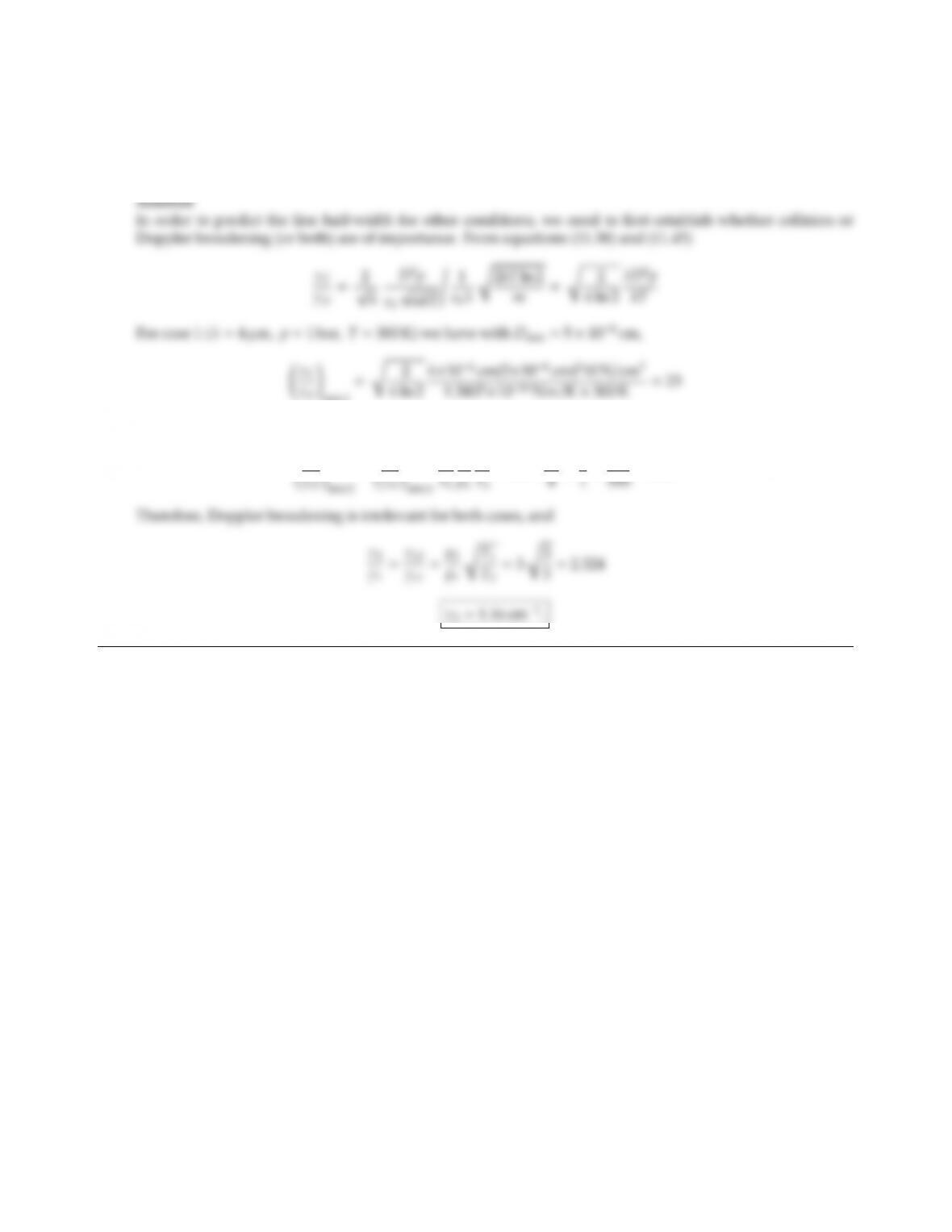CHAPTER 11 295
11.7 A certain gas has two important vibration-rotation bands centered at 4 µm and 10 µm. Measurements of
spectral lines in the 4 µm band (taken at 300 K and 1 bar =105N/m2) indicate a half-width of γη=0.5 cm1.
Predict the half-width in the 10µm band for the gas at 500K, 3bar. (The diameter of the gas molecules is
known to be between 5 Å <D<40 Å).
Thus, for case 1 collision broadening dominates. For case 2
γC
= γC
λ2
p2
T1
=23 ×10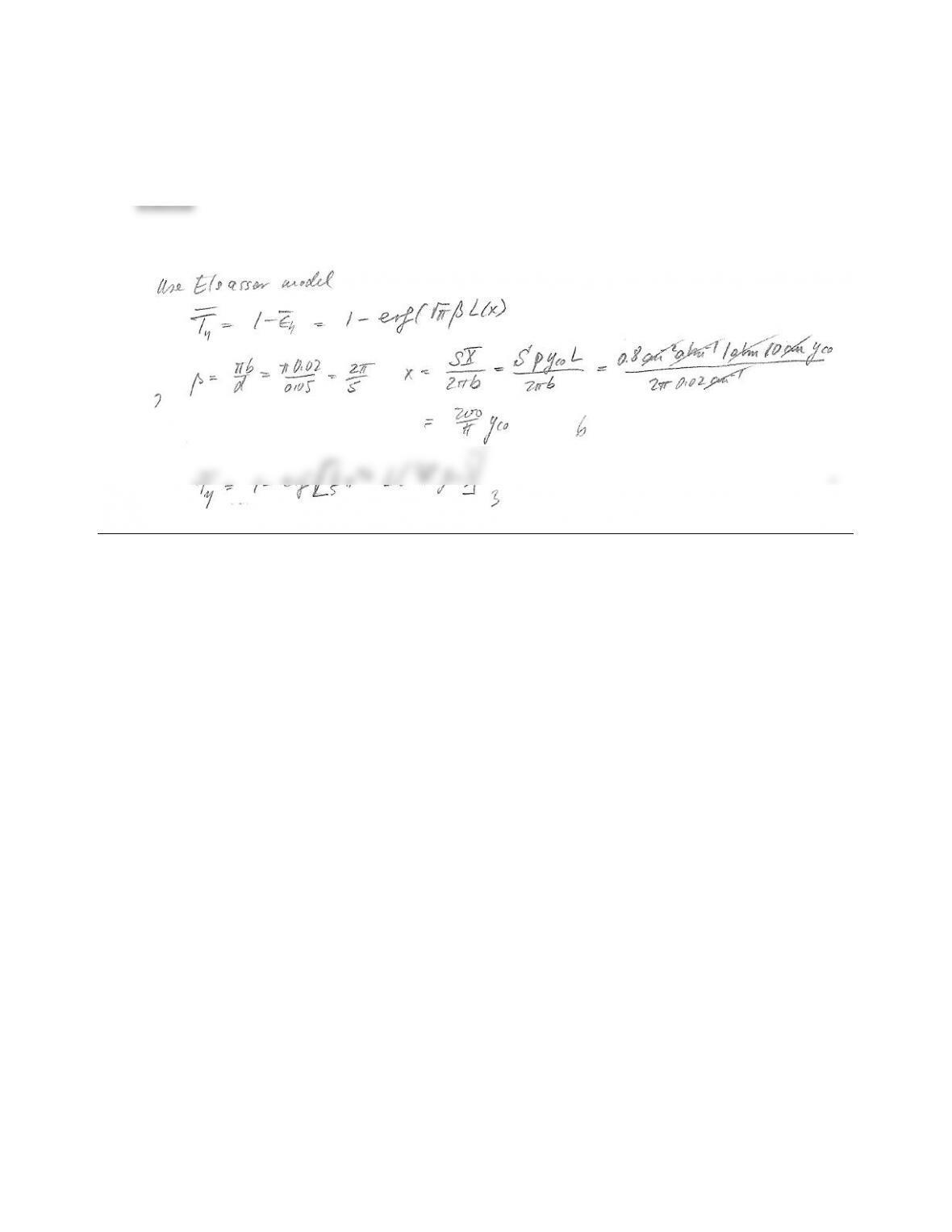11.8 It is desired to measure the volume fraction of CO in a hot gas by measuring the transmissivity of a 10 cm long
column, using a blackbody source and a detector responsive around 4.7 µm. The conditions in the column
are 1000 K, 1 atm, and properties for CO around 4.7 µm are known to be S=0.8 cm2atm1,γ=0.02 cm1,
and d=0.05 cm1. Give an expression relating measured transmissivity to CO volume fraction.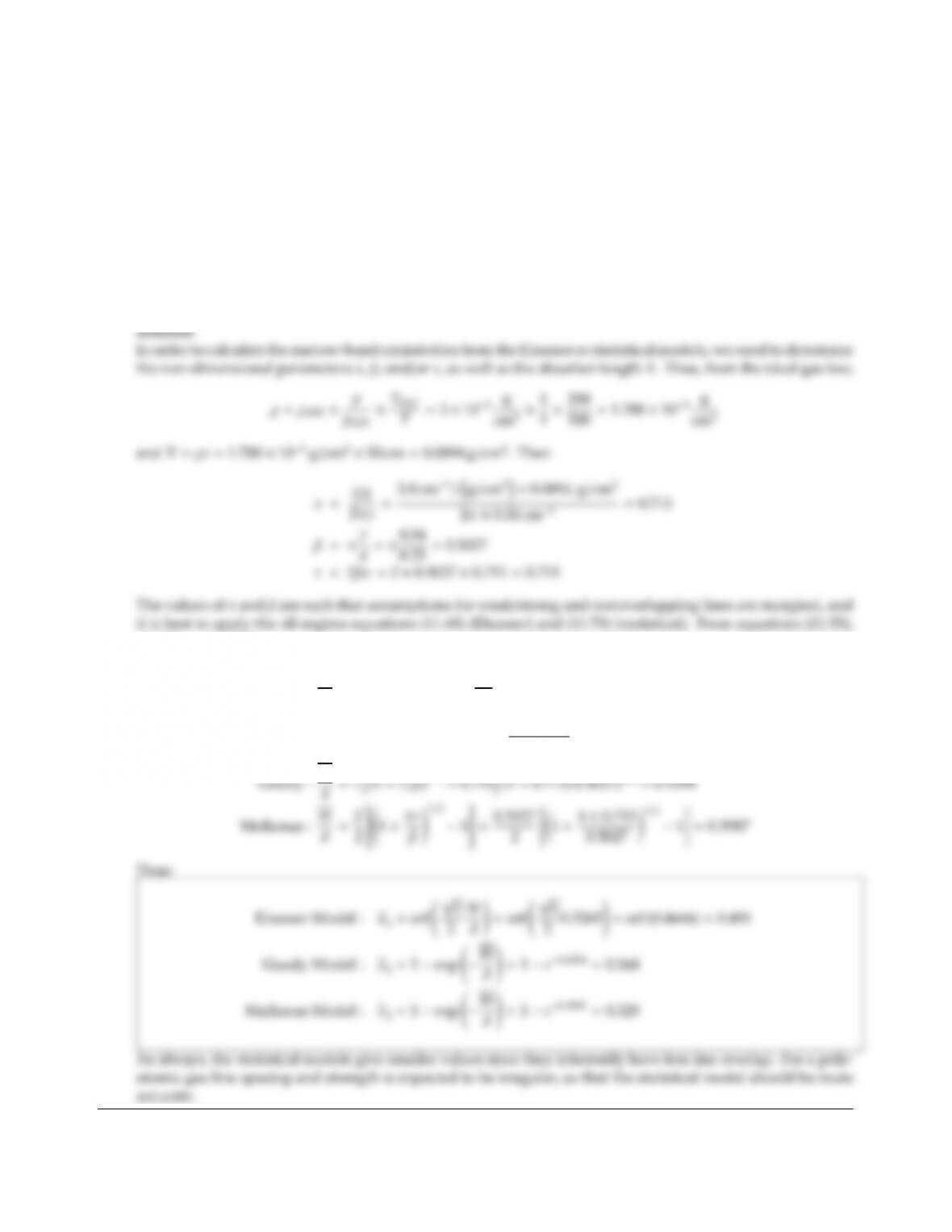CHAPTER 11 297
11.9 A polyatomic gas has an absorption band in the infrared. For a certain small wavelength range to the
following is known:
Average line-half width: 0.04 cm1,
Average integrated absorption coecient: 2.0×104cm1/(g/m2),
Average line spacing: 0.25 cm1,
The density of the gas at STP is 3 ×103g/cm3.
For a 50 cm thick gas layer at 500 K and 1 atm calculate the local mean spectral emissivity using
(a) the Elsasser model,
(b) the statistical model.
Which result can be expected to be more accurate?
(11.78) and (11.79)
Elsasser : W
d=2βL(x)2βx"1+πx
25/4#2/5
=2×0.5027 ×0.711 "1+π×0.711
25/4#2/5
=0.5265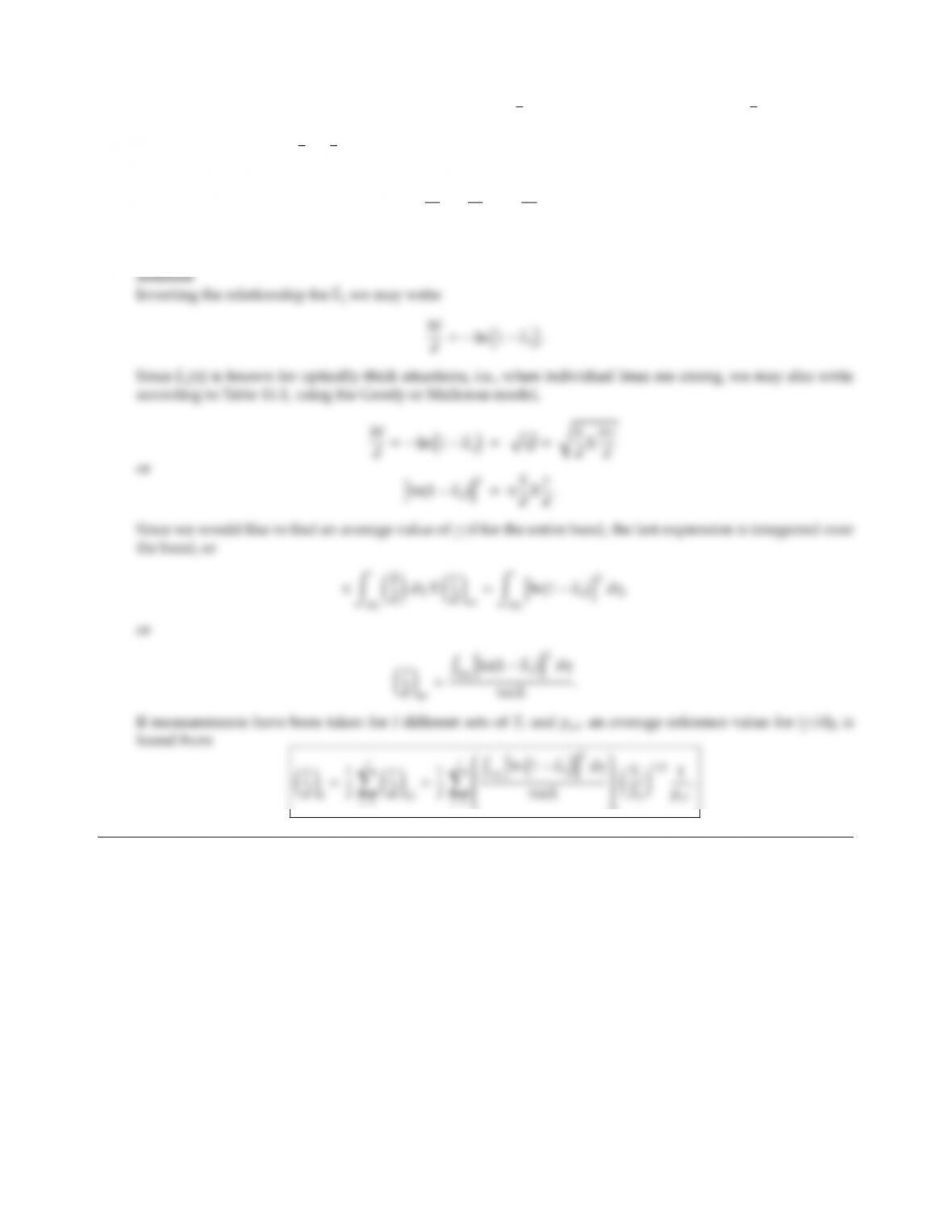11.10 Consider a gas for which the model is applicable, i.e., ǫη=1exp(Wη/d). To predict ǫηfor arbitrary
situations, a band-averaged (or constant) value for γη/dmust be known. Experimentally available are values
for α=Rη(Sη/d)dηand ǫη=ǫη(η) (for optically thick situations) for given peand T. It is also known that
γη
dγη
d0peT0
T1/2
.
Outline how an average value for (γη/d)0can be found.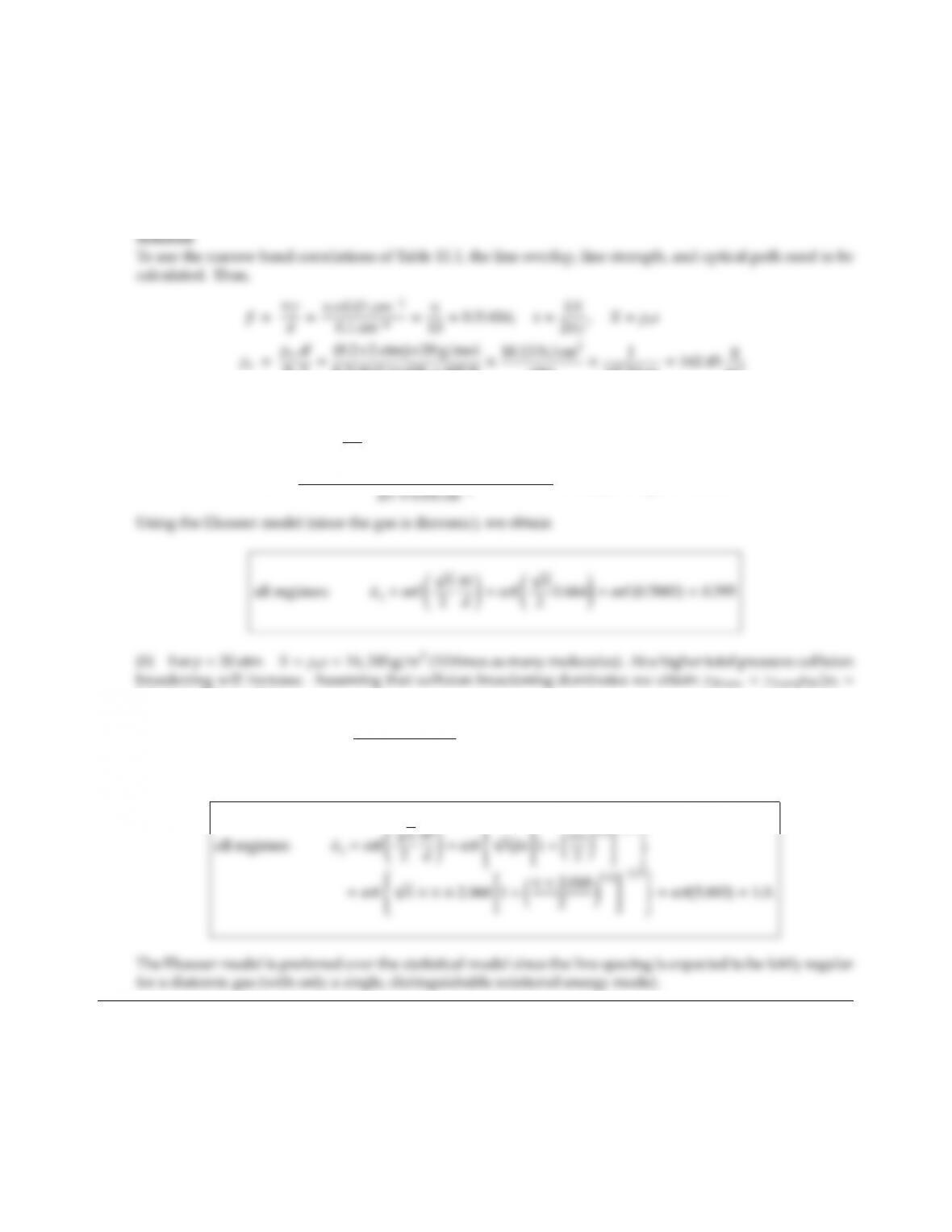CHAPTER 11 299
11.11 The following is known for a gas mixture at 600K and 2atm total pressure and in the vicinity of a certain
spectral position: The gas consists of 80% (by volume) N2and 20% of a diatomic absorbing gas with a
molecular weight of 20 g/mol, a mean line half-width γ=0.01 cm1, a mean line spacing of d=0.1 cm1,
and a mean line strength of S=8×105cm2/(g/m3). (a) For a gas column 10 cm thick determine the mean
spectral emissivity of the gas. (b) What happens if the pressure is increased to 20atm? (Since no broadening
parameters are known you may assume the eective broadening pressure to be equal to the total pressure).
RuT=(0.2×2 atm)×20 g/mol
8.3144 J/mol K ×600 K ×10.13 N/cm2
atm ×J
102Ncm =162.45 g
m3
(a)
X=162.45 g
m310 cm =1624.5 cm g/m3,
x=8×105cm2/(g/m3)×1624.5 cm(g/m3)
β=π, x=8×105×16,245
2π×0.1=2.068 (unchanged) and τ=13.012.
Thus: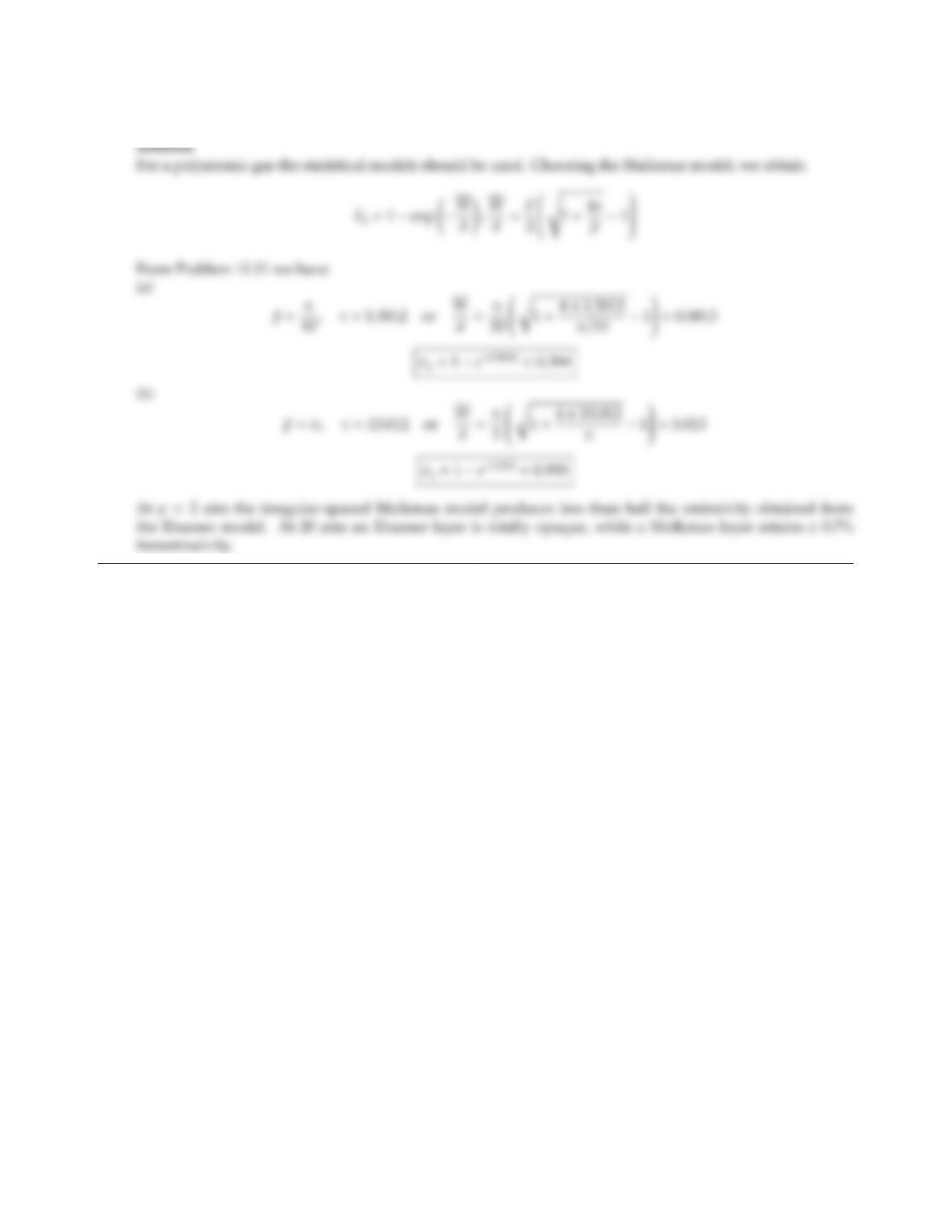11.12 Repeat Problem 11.11 for a four-atomic gas.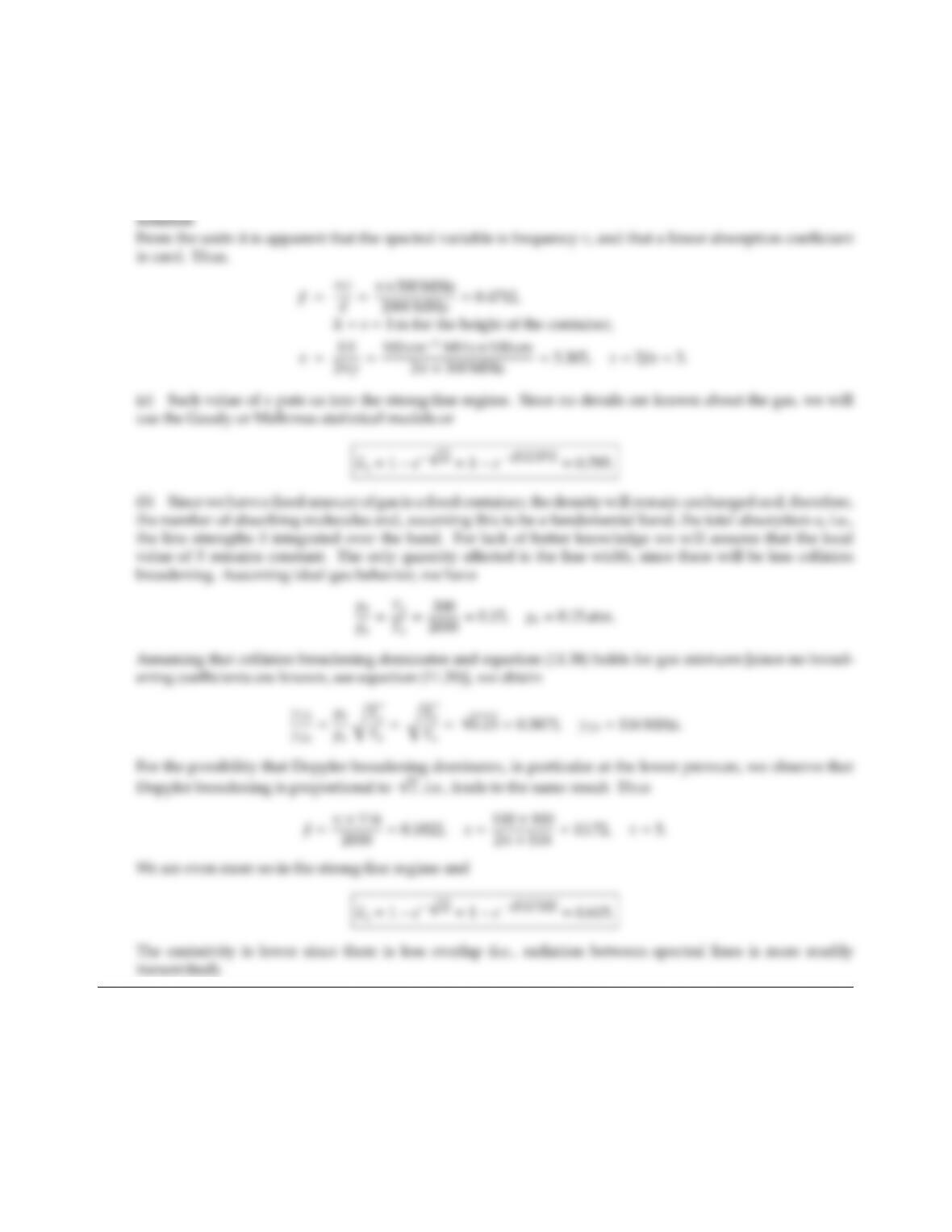CHAPTER 11 301
11.13 1 kg of a gas mixture at 2000 K and 1 atm occupies a container of 1 m height. The gas consists of 70% nitrogen
(by volume) and of 30% an absorbing species. It is known that, at a certain spectral location, the line half-
width is γ=300 MHz, the mean line spacing is d=2000 MHz, and the line strength is S=100 cm1MHz. (a)
Calculate the mean spectral emissivity under these conditions. (b) What will happen to the emissivity if the
sealed container is cooled to 300 K?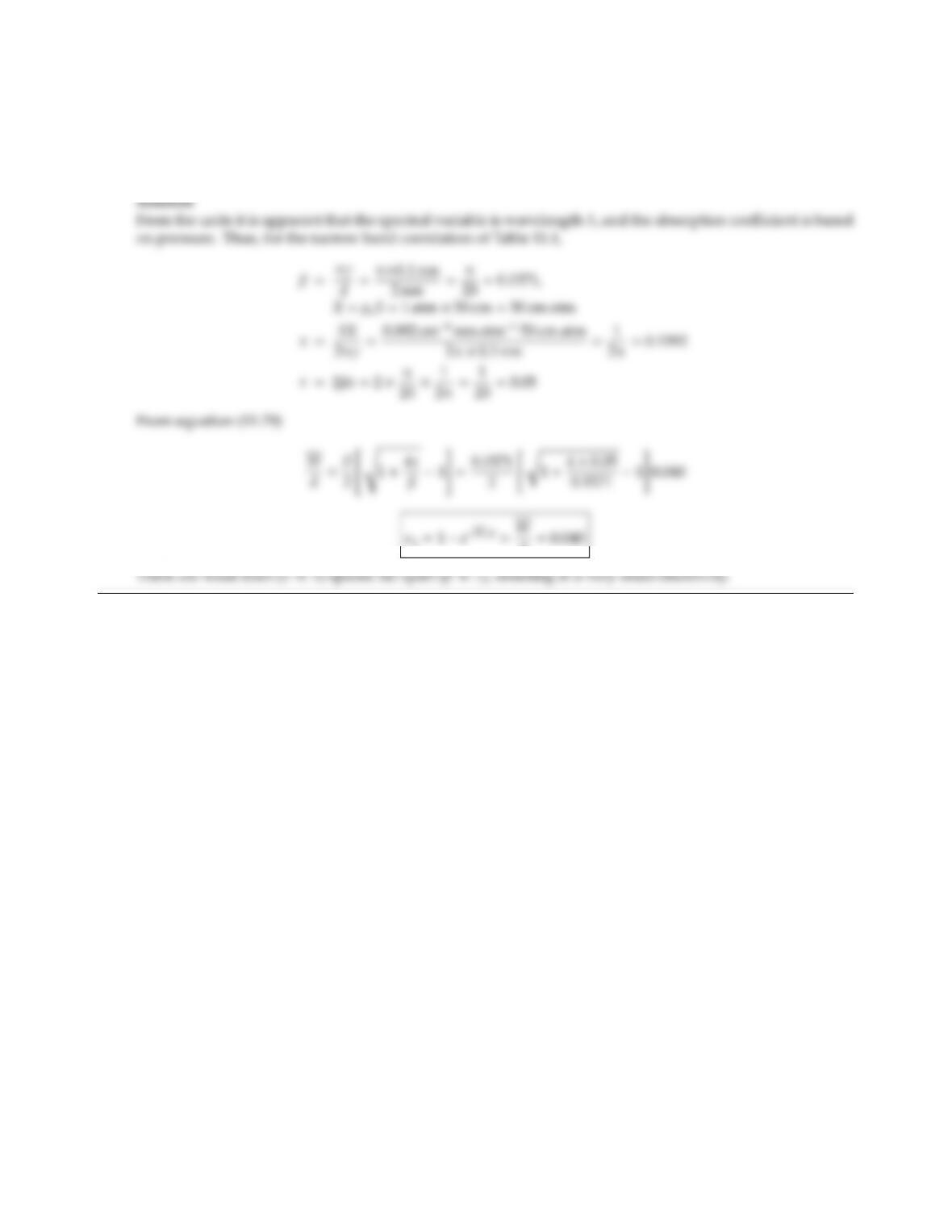11.14 A 50 cm thick layer of a pure gas is maintained at 1000K and 1 atm. It is known that, at a certain spectral
location, the mean line half-width is γ=0.1 nm, the mean line spacing is d=2 nm, and the mean line strength
is S=0.002 cm1nm atm1=2×1010 atm1. What is the mean spectral emissivity under these conditions?
(1 nm =109m.)
d=0.040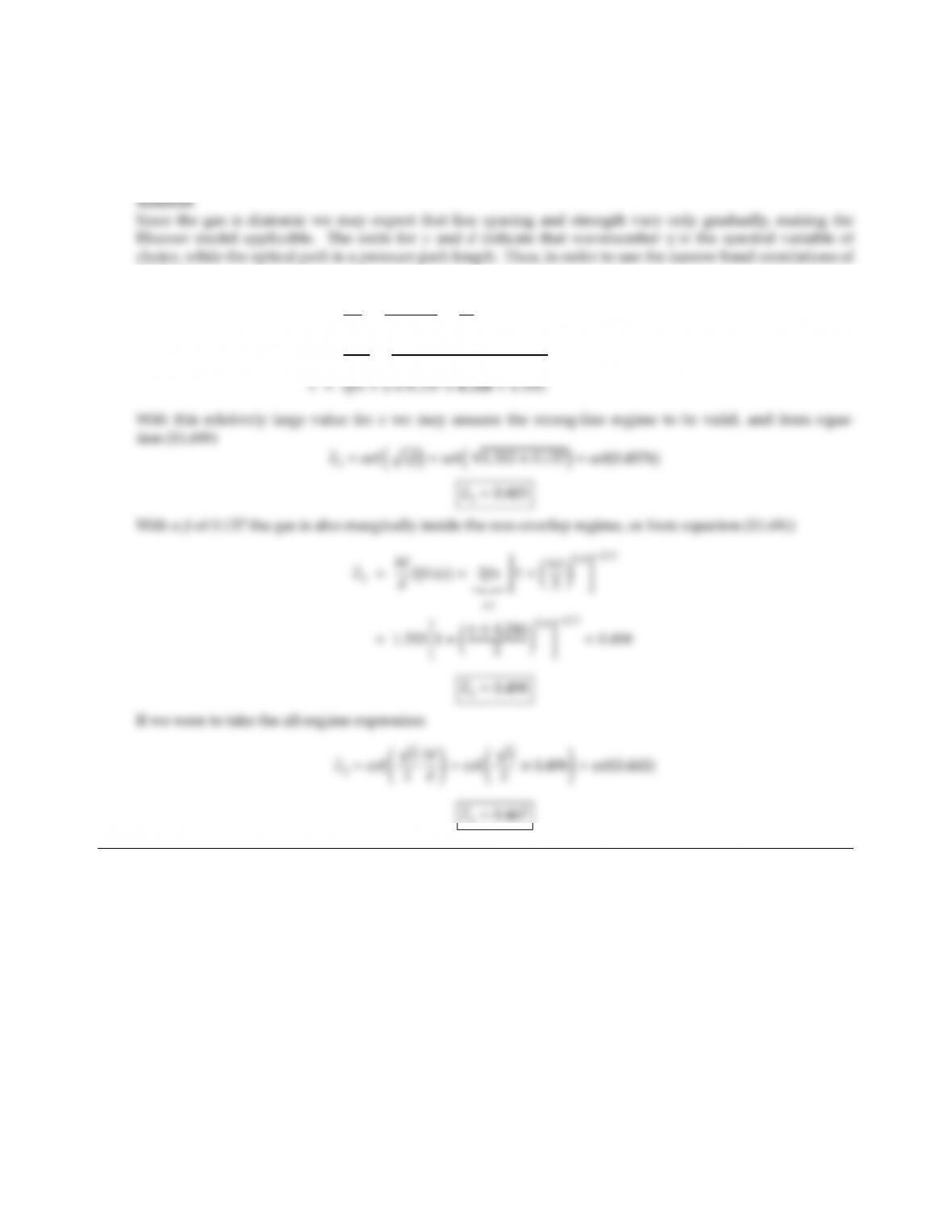CHAPTER 11 303
11.15 The following data for a diatomic gas at 300 K and 1 atm are known: The mean line spacing is 0.6 cm1and the
mean line half-width is 0.03 cm1; the mean line strength (=integrated absorption coecient) is 0.8 cm2atm1
(based on a pressure absorption coecient). Calculate the mean spectral emissivity for a path length of 1 cm.
In what band approximation is the optical condition?
Table 11.1,
β=πγ
d=π×0.03
0.6=π
20 =0.157,X=pL =1 cm atm,
x=SX
2πγ =0.8 cm2atm11 cm atm
2π×0.03 cm1=4.244,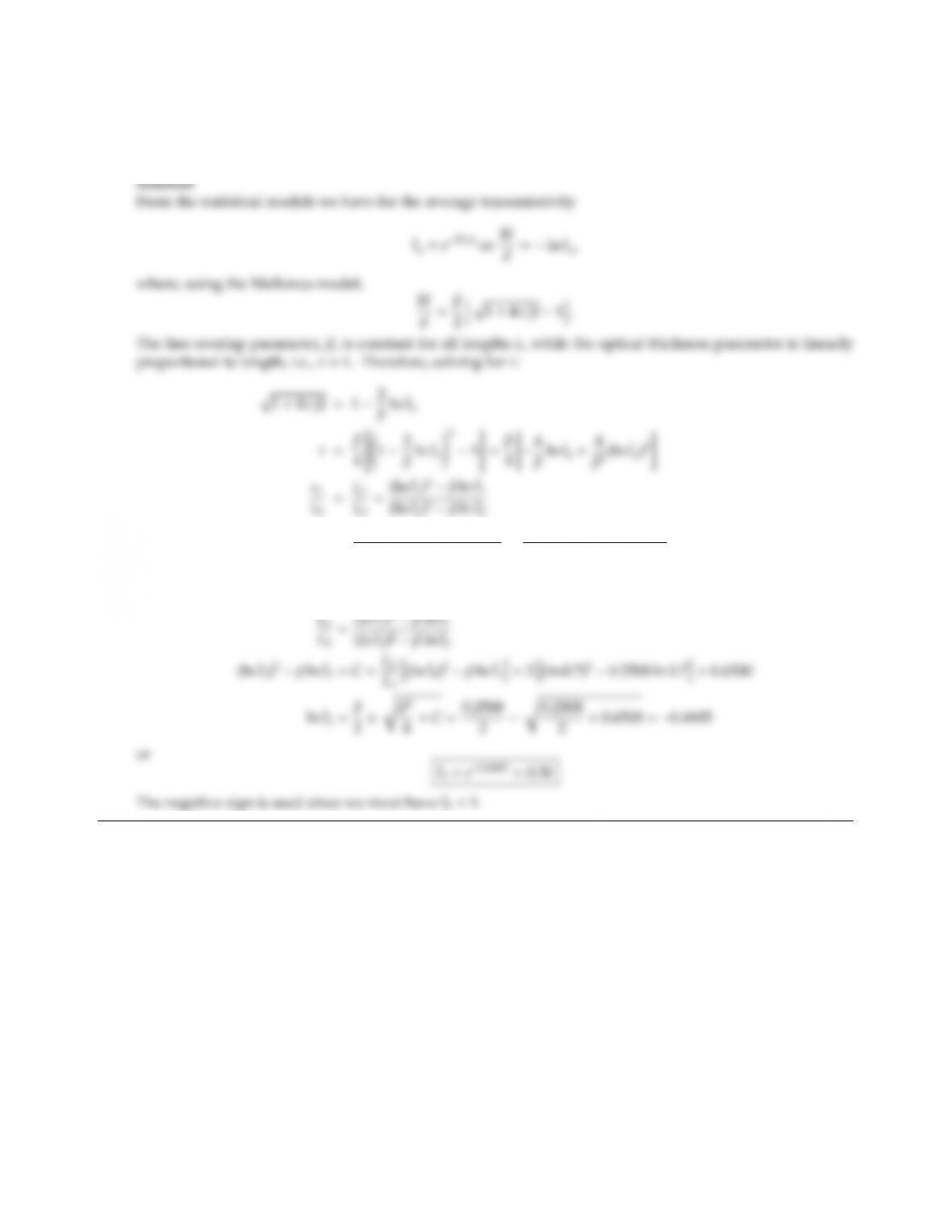11.16 The average narrow band transmissivity of a homogeneous gas mixture has, at a certain wavenumber η, been
measured as 0.70 for a length of 10cm, and as 0.58 for a length of 20 cm. What is the expected transmissivity
for a gas column of 30 cm length, assuming the Malkmus model to hold?
β=(ln ¯
t1)2(L1/L2)(ln ¯
t2)2
ln ¯
t1(L1/L2) ln ¯
t2
=(ln 0.7)20.5(ln 0.58)2
ln 0.70.5 ln 0.58 =0.2508
This formula applies for any two lengths. Thus,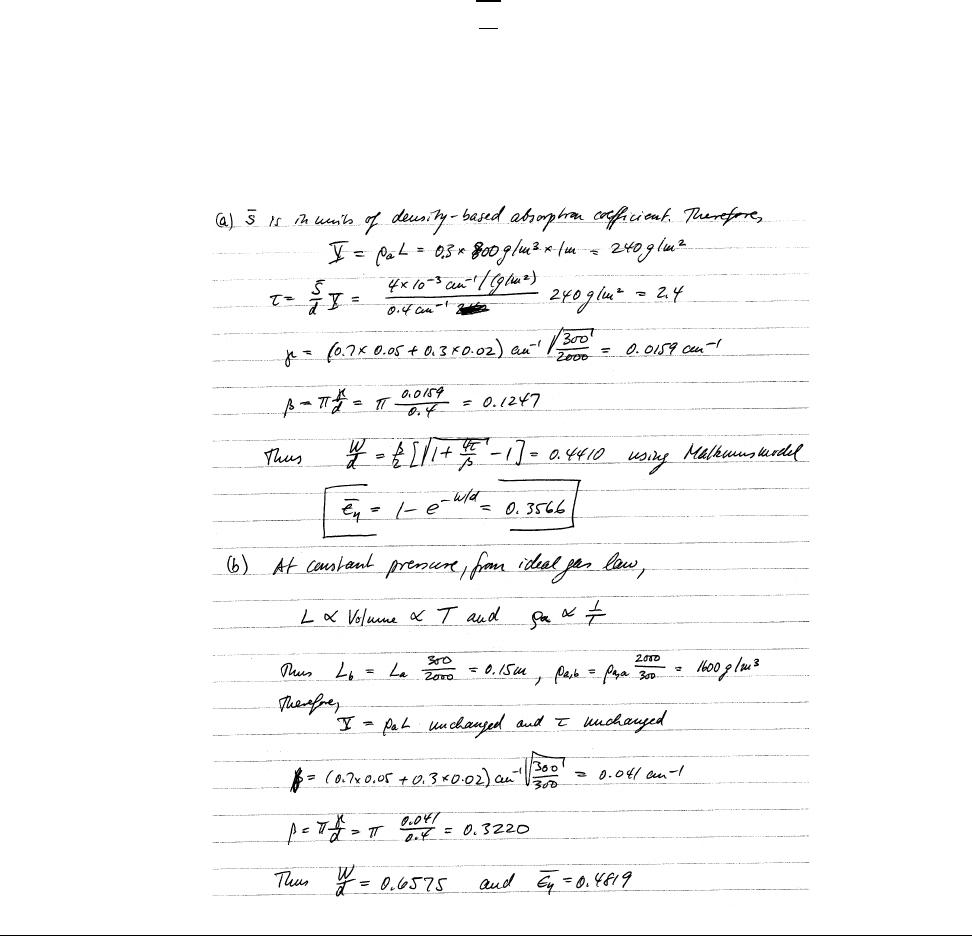CHAPTER 11 305
11.17 1 kg of a gas mixture at 2000 K and 1 atm occupies a container of 1 m height. The gas consists of 70% nitrogen
(by volume) and 30% of an absorbing species. It is known that, at a certain spectral location, the nitrogen-
broadening line half-width at STP (1atm and 300K) is γn0=0.05 cm1, the self-broadening line half-width is
γa0=0.02 cm1, the mean line spacing is d=0.4 cm1, and the density and mean line strength (for the given
mixture conditions) are ρ=0.800 kg/m3and ¯
S=4×103cm1/(g/m2), respectively. Under these conditions
collision broadening is expected to dominate.
(a) Calculate the mean spectral emissivity based on the height of the container.
(b) What will happen to the emissivity if the sealed container is cooled to 300 K at constant pressure (with
fixed container cross-section and sinking top end)?
Note: the mean line intensity is directly proportional to the number of molecules of the absorbing gas and
otherwise constant. The line half-width is given by
γ=[γn0pn+γa0pa]rT0
T(pin atm,T0=300 K),
where pnand paare partial pressures of nitrogen and absorbing species.
Solution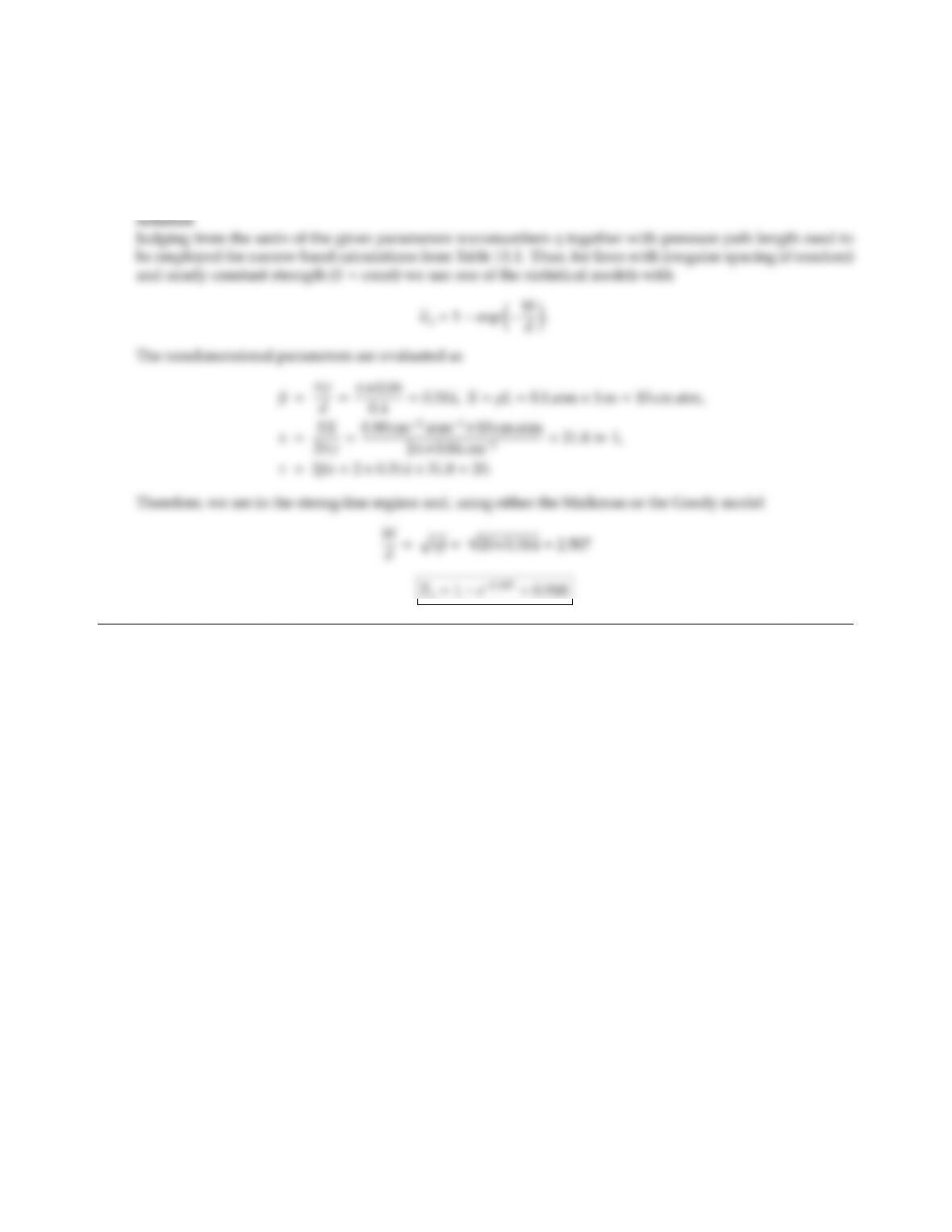11.18 A certain gas is known to behave almost according to the rigid-rotor/harmonic-oscillator model, resulting in
gradually changing line strengths (with wavenumber) and somewhat irregular line spacing. Calculate the
local mean emissivity for a 1 m thick layer of the gas at 0.1 atm pressure. In the wavelength range of interest, it
is known that the integrated absorption coecient is equal to 0.80 cm2atm1, the line half-width is 0.04 cm1
and the average line spacing is 0.40 cm1.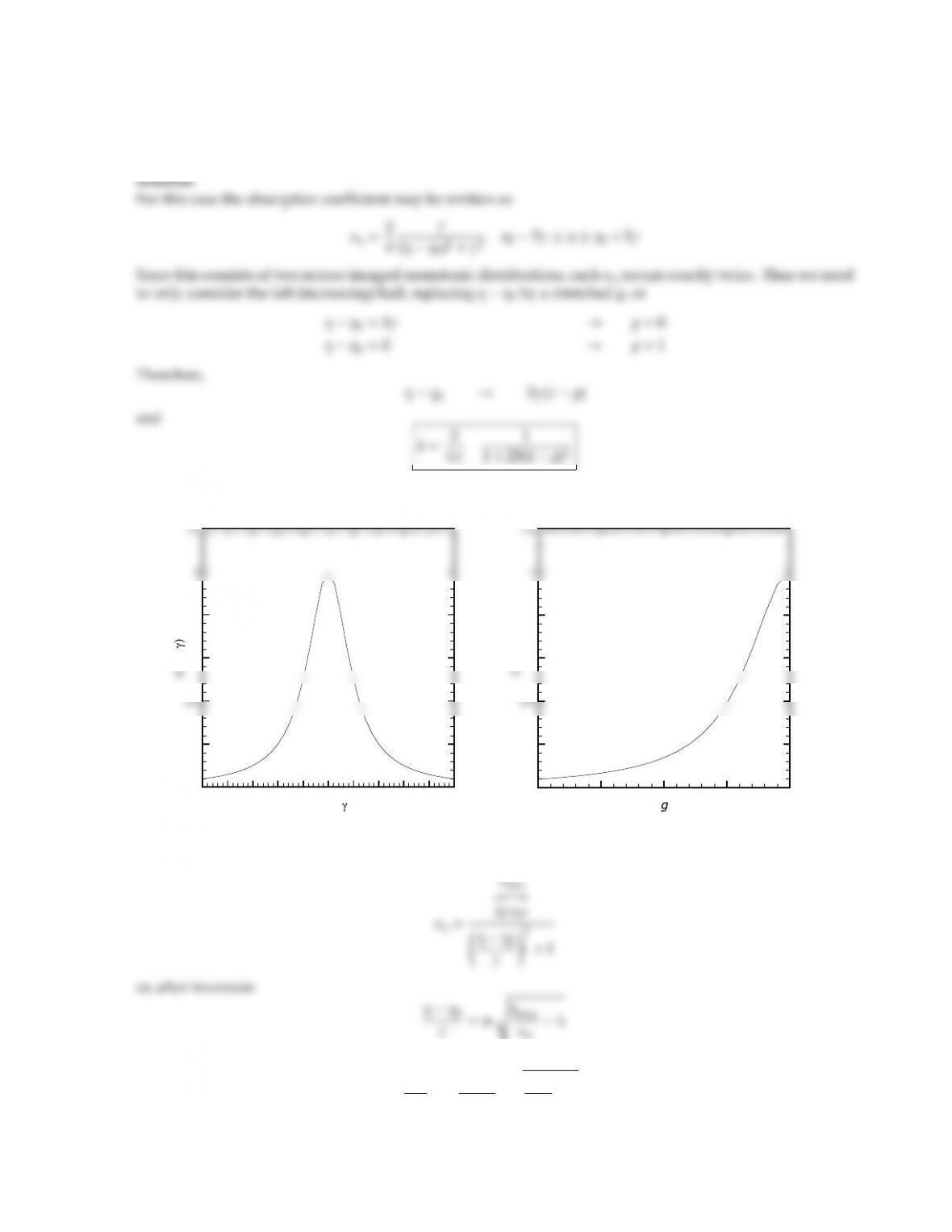CHAPTER 11 307
11.19 A narrow band of a certain absorbing gas contains a single spectral line of Lorentz shape at its center. For a
narrow band width of η=10γ, determine the corresponding reordered kvs. 1distribution.
Hint: this can be achieved without a lot of math.
(η›η0)/
›5 ›4 ›3 ›2 ›1 0 1 2 3 4 5
0
0.2
0.6
0.8
0 0.25 0.5 0.75 1
0
0.2
0.6
0.8
Masochists can, of course, also solve this problem mathematically:
Dierentiating
dη
dκη
=γkmax
2κ2
η,skmax
κη1,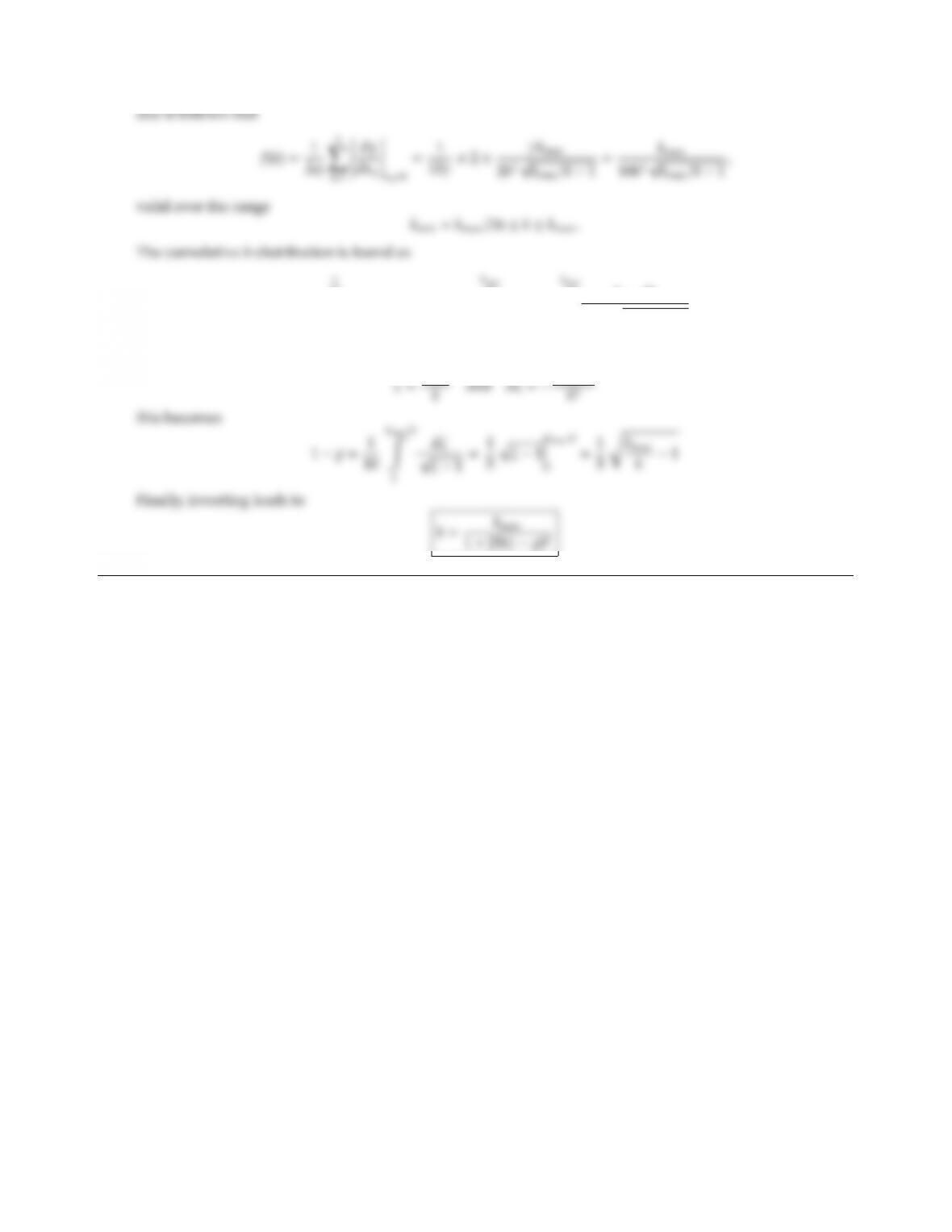1=
kmin
f(k)dk or 1 1=
k
f(k)dk =
k
kmaxdk
10k2kmax/k1.
Using

## Trusted by Thousands ofStudents

Here are what students say about us.